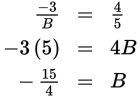# SAT Math Multiple Choice Question 794: Answer and Explanation

### Test Information

Question: 794

14.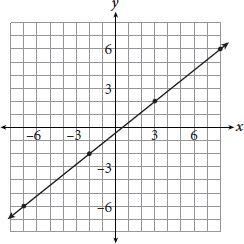If the equation of the line shown on the graph is written in standard form, Ax + By = C, and A = 3, what is the value of B?

• A. –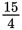• B. –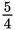• C.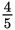• D. 15

Explanation:

A

Difficulty: Hard

Category: Heart of Algebra / Linear Equations

Strategic Advice: Whenever there is more than one strategy that could get you to the answer, always consider which one will get you there faster. Here, you could use two points on the line to write its equation and then convert the equation to standard form, or you can convert standard form to slope-intercept form and compare the slope to that of the line in the graph. Use whichever method will get you to the answer quicker.

Getting to the Answer: Rewrite Ax + By = C in slope-intercept form: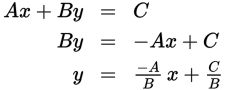The slope of the line is the coefficient of x, which is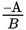. Now, find the slope of the line shown in the graph by counting the rise and the run from one point to the next; the slope is.

You're given that A = 3, so=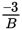. Set this quantity equal toand cross-multiply to solve for B: